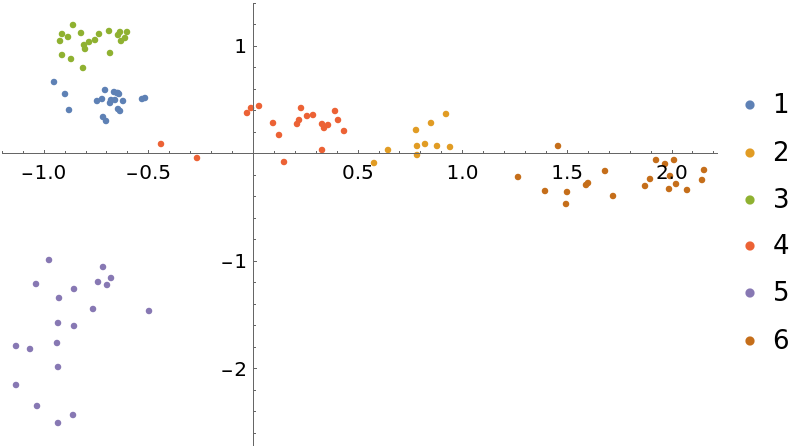#Function Repository Resource:

# MultidimensionalScaling

Reduce a matrix of real values to low dimension using the principal coordinates analysis method

Contributed by: Daniel Lichtblau
 ResourceFunction["MultidimensionalScaling"][vecs,dim] uses principal coordinates analysis to find a "best projection" of vecs to dimension dim. ResourceFunction["MultidimensionalScaling"][vecs] projects vecs to two dimensions.

## Details

Multidimensional Scaling (MDS) is a standard method for reducing dimension of a set of numerical vectors. It is related to the "LatentSemanticAnalysis" and "PrincipalComponentsAnalysis" methods of DimensionReduce.
ResourceFunction["MultidimensionalScaling"] gives an optimal reduction according to a certain Euclidean measure.
Given a set of n vectors, ResourceFunction["MultidimensionalScaling"] will create a list of all pairwise distances, that is, an n×n dense matrix of real values. It is thus not recommended to use this when n is large.

## Examples

### Basic Examples (1)

Reduce the dimension of some vectors:

 In:=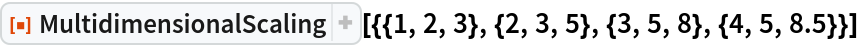Out=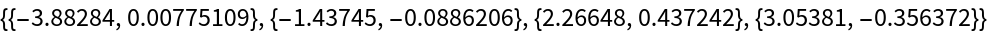### Scope (2)

Create and visualize random 3D vectors:

 In:=Out=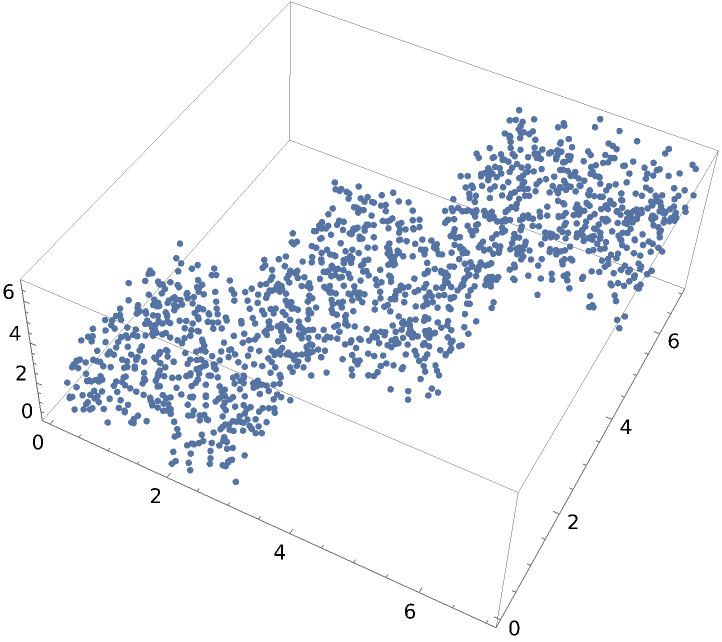Visualize this dataset reduced to two dimensions:

 In:=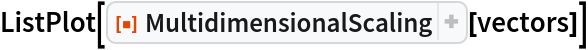Out=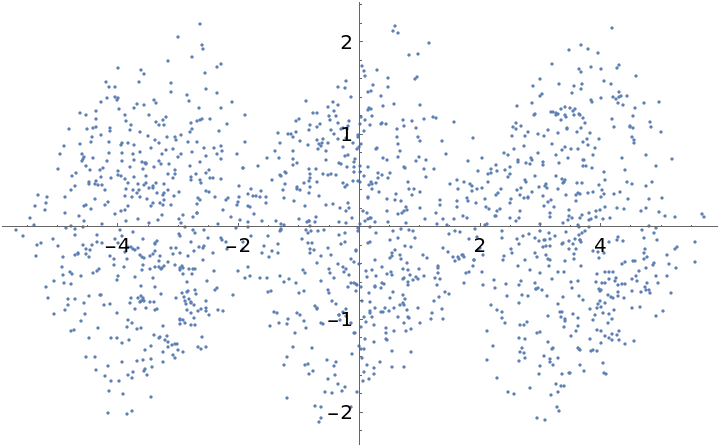MultidimensionalScaling will reduce to any dimension that is no larger than the input dimension. Here we create data in ten dimensional space, and visualize in three dimensions:

 In:=In:=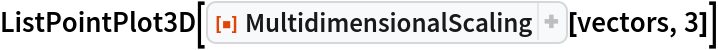Out=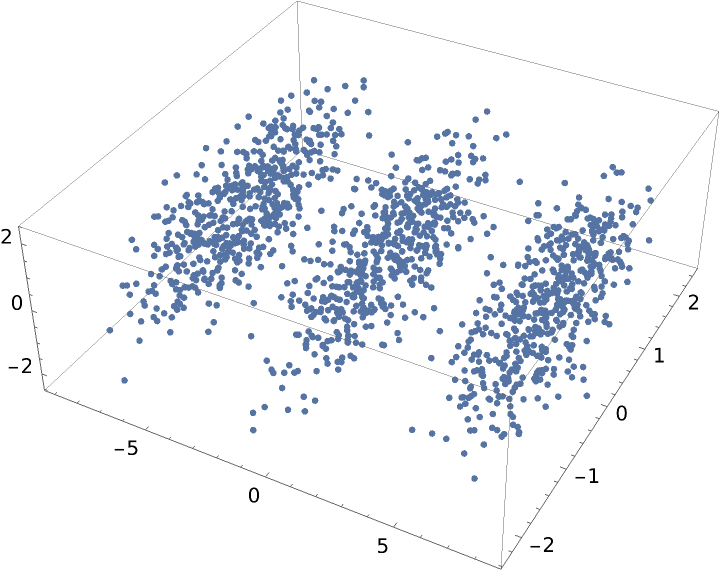### Properties and Relations (8)

As is done in the reference page for DimensionReduce, load the Fisher iris dataset from ExampleData:

 In:=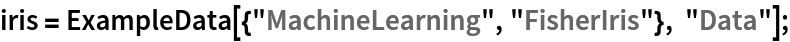Reduce the dimension of the features:

 In:=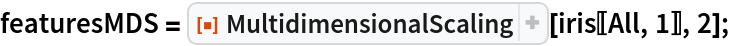Group the examples by their species:

 In:=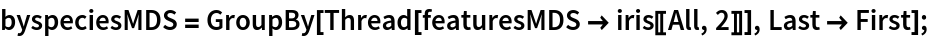Visualize the reduced dataset:

 In:=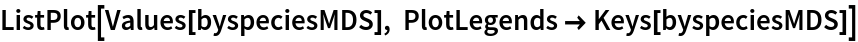Out=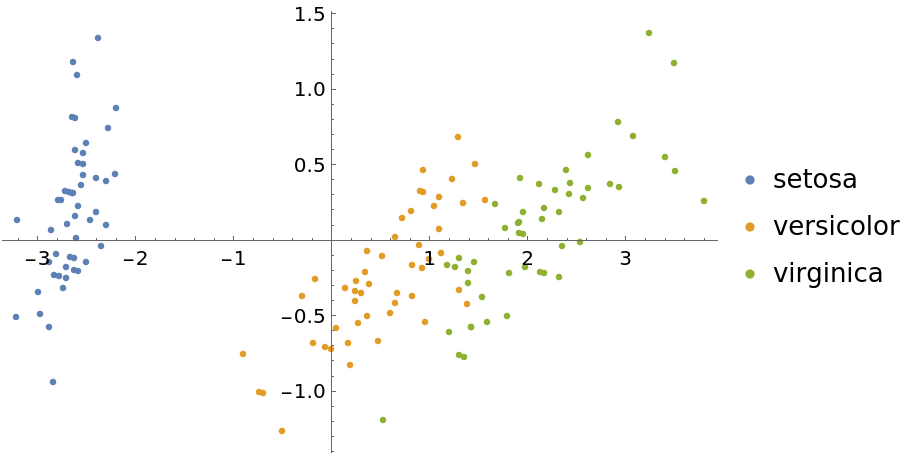Now show some DimensionReduce methods for this same dataset. First we use the "PrincipalComponentsAnalysis" method:

 In:=Out=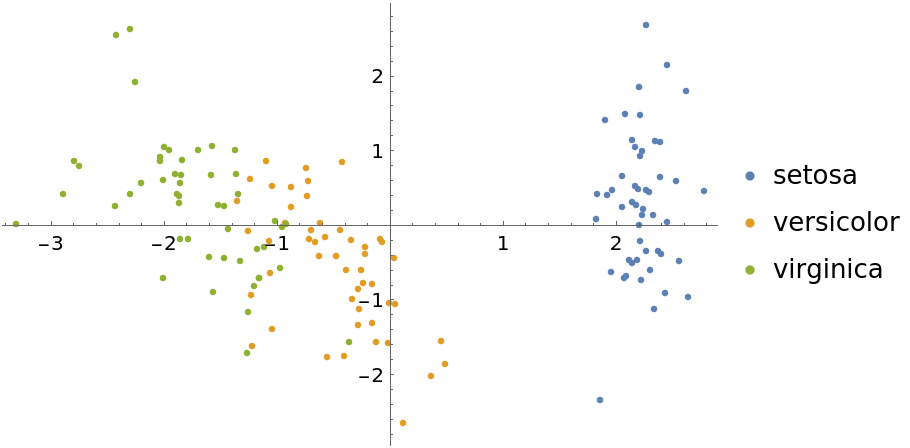Use the "TSNE" method:

 In:=Out=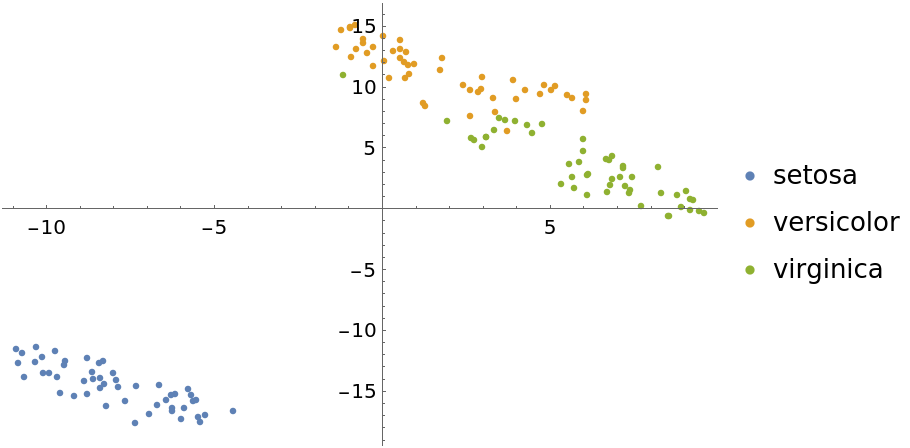Visualize with the "LatentSemanticAnalysis" method:

 In:=Out=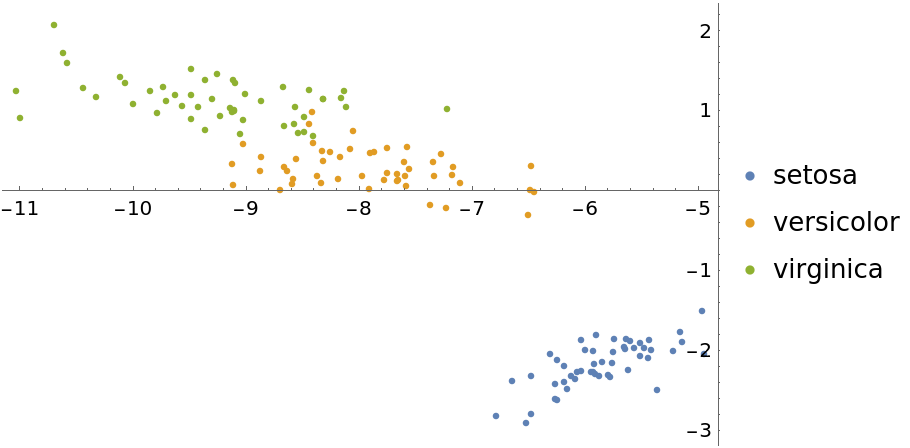The "LatentSemanticAnalysis" method can be attained directly using SingularValueDecomposition:

 In:=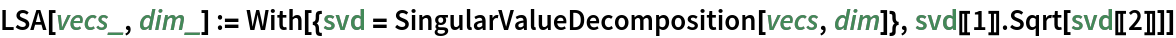In:=Out=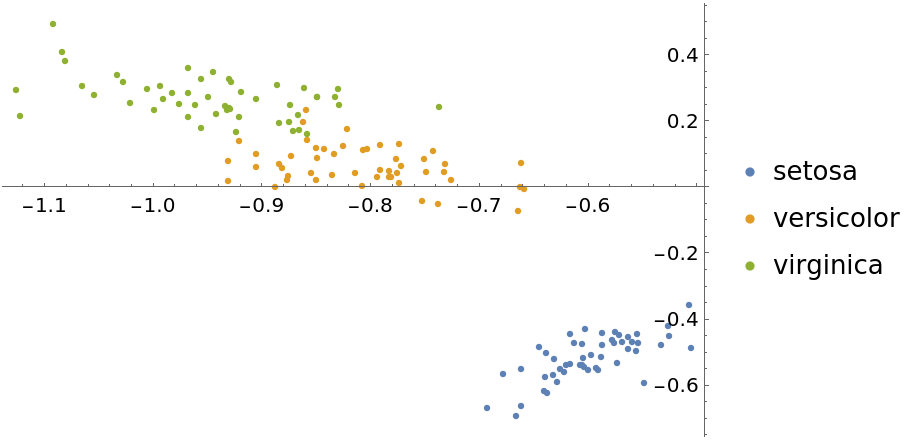### Neat Examples (6)

Illustrate multidimensional scaling on textual data using several popular literature texts from ExampleData:Break each text into chunks of equal string length:Find the most common words across all texts:Create common word frequency vectors for each chunk:Weight the frequency vectors using the log-entropy method:Show the result of multidimensional scaling in two dimensions, grouping text chunks by position of title in the list of text names:

 In:=Out=